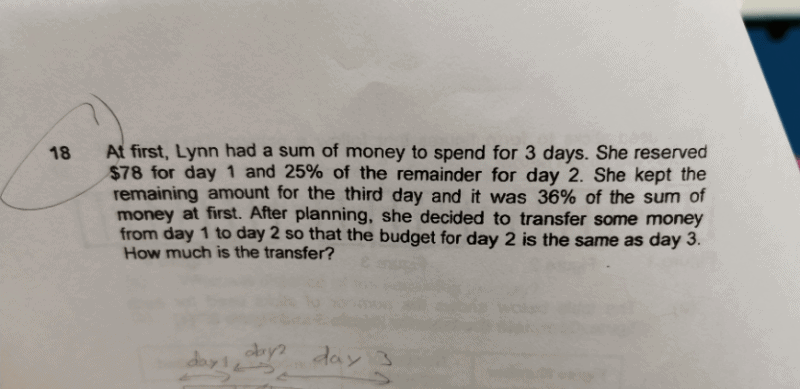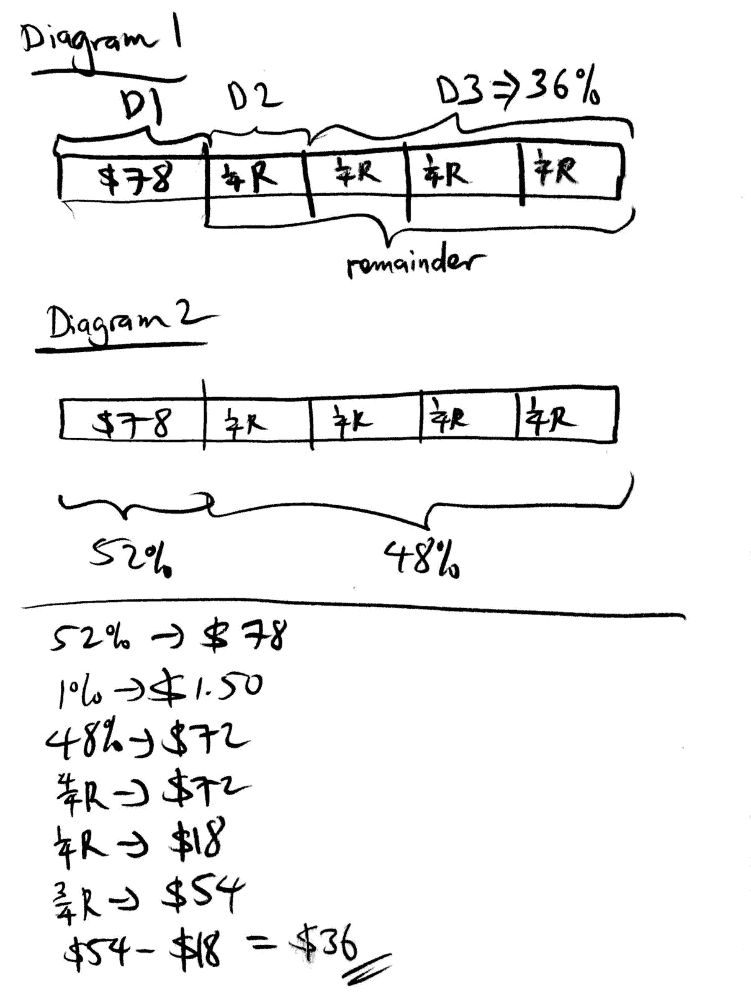# QuestionHi, need help on this qns.

ThanksHi Bunny27, let me try to help you out with this question. I’ll provide the solution and some explanation too.

Taking a look at Diagram 1, we see that 3/4 of the remainder of the money that was reserved on Day 3, is equivalent to 36% of the total sum of money at first. That means, referring to Diagram 2, we can see that 4/4 of the remainder is equivalent to 48% of the total sum of money at first.

Since Day 2 and Day 3 makes up 48% of the total sum of money at first, it means, in Day 1, it makes up 52% of the total sum of money at first. 52% represents \$78. We find out that 1% of the total sum of money at first would be \$1.50. Hence, 48% would be \$72.

4/4 of the remainder is also \$72, so 1/4 of the remainder is \$18.

We work out 3/4 of the remainder (Day 3) to be \$54.

\$54 – \$18 = \$36

Hence, in order for Day 2 to be same as Day 3, \$36 needs to be transferred.

This question uses the Part-Whole Model. It is also known as the Long Box Method, where the students are tested on their skills for model drawing and model manipulation. Very common question in Paper 2 of the Mathematics Exam Paper. Will be happy to share more with you .

Hope this solution provided above helps!

Feel free to PM or text me (:

http://www.futureachievers.com.sg

9114 3397

0 Replies 2 Likes